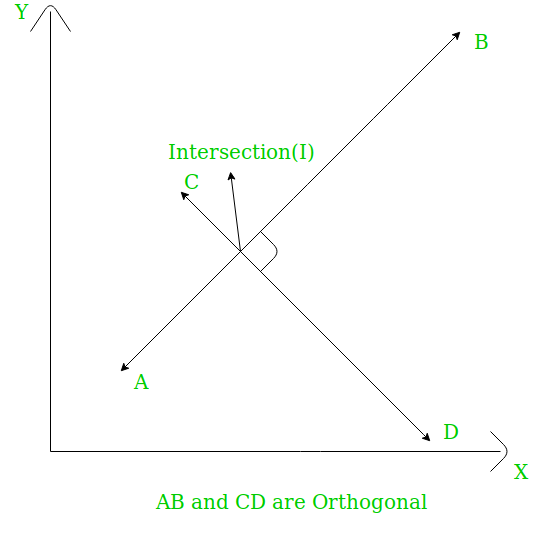Related Articles

# Check whether two straight lines are orthogonal or not

• Difficulty Level : Basic
• Last Updated : 11 May, 2021

Given two line segments AB and CD having A(x1, y1), B(x2, y2), C(x3, y3) and D(x4, y4). The task is to check whether these two lines are orthogonal or not. Two lines are called orthogonal if they are perpendicular at the point of intersection.Attention reader! Don’t stop learning now. Get hold of all the important mathematical concepts for competitive programming with the Essential Maths for CP Course at a student-friendly price. To complete your preparation from learning a language to DS Algo and many more,  please refer Complete Interview Preparation Course.

Examples:

```Input: x1 = 0, y1 = 3, x2 = 0, y2 = -5
x3 = 2, y3 = 0, x4 = -1, y4 = 0
Output: Yes

Input:  x1 = 0, y1 = 4, x2 = 0, y2 = -9
x3 = 2, y3 = 0, x4 = -1, y4 = 0
Output: Yes```

Approach: If the slopes of the two lines are m1 and m2 then for them to be orthogonal we need to check if:

• Both lines have infinite slope then answer is no.
• One line has infinite slope and if other line has 0 slope then answer is yes otherwise no.
• Both lines have finite slope and their product is -1 then the answer is yes.

Below is the implementation of the above approach:

## C++

 `// C++ implementation of above approach``#include ``using` `namespace` `std;` `// Function to check if two straight``// lines are orthogonal or not``bool` `checkOrtho(``int` `x1, ``int` `y1, ``int` `x2, ``int` `y2,``                ``int` `x3, ``int` `y3, ``int` `x4, ``int` `y4)``{` `    ``int` `m1, m2;` `    ``// Both lines have infinite slope``    ``if` `(x2 - x1 == 0 && x4 - x3 == 0)``        ``return` `false``;` `    ``// Only line 1 has infinite slope``    ``else` `if` `(x2 - x1 == 0) {` `        ``m2 = (y4 - y3) / (x4 - x3);` `        ``if` `(m2 == 0)``            ``return` `true``;``        ``else``            ``return` `false``;``    ``}` `    ``// Only line 2 has infinite slope``    ``else` `if` `(x4 - x3 == 0) {` `        ``m1 = (y2 - y1) / (x2 - x1);` `        ``if` `(m1 == 0)``            ``return` `true``;``        ``else``            ``return` `false``;``    ``}` `    ``else` `{``        ``// Find slopes of the lines``        ``m1 = (y2 - y1) / (x2 - x1);``        ``m2 = (y4 - y3) / (x4 - x3);` `        ``// Check if their product is -1``        ``if` `(m1 * m2 == -1)``            ``return` `true``;``        ``else``            ``return` `false``;``    ``}``}` `// Driver code``int` `main()``{``    ``int` `x1 = 0, y1 = 4, x2 = 0, y2 = -9;``    ``int` `x3 = 2, y3 = 0, x4 = -1, y4 = 0;` `    ``checkOrtho(x1, y1, x2, y2, x3, y3, x4, y4) ? cout << ``"Yes"``                                               ``: cout << ``"No"``;` `    ``return` `0;``}`

## Java

 `//Java implementation of above approach` `import` `java.io.*;` `class` `GFG {``    ` `    ``// Function to check if two straight``    ``// lines are orthogonal or not``    ``static` `boolean` `checkOrtho(``int` `x1, ``int` `y1, ``int` `x2, ``int` `y2,``                    ``int` `x3, ``int` `y3, ``int` `x4, ``int` `y4)``    ``{` `        ``int` `m1, m2;``    ` `        ``// Both lines have infinite slope``        ``if` `(x2 - x1 == ``0` `&& x4 - x3 == ``0``)``            ``return` `false``;` `        ``// Only line 1 has infinite slope``        ``else` `if` `(x2 - x1 == ``0``)``        ``{``            ``m2 = (y4 - y3) / (x4 - x3);``            ``if` `(m2 == ``0``)``                ``return` `true``;``            ``else``                ``return` `false``;``        ``}` `        ``// Only line 2 has infinite slope``        ``else` `if` `(x4 - x3 == ``0``)``        ``{``             ``m1 = (y2 - y1) / (x2 - x1);``            ``if` `(m1 == ``0``)``                ``return` `true``;``            ``else``                ``return` `false``;``        ``}` `        ``else``        ``{``            ``// Find slopes of the lines``            ``m1 = (y2 - y1) / (x2 - x1);``            ``m2 = (y4 - y3) / (x4 - x3);` `            ``// Check if their product is -1``            ``if` `(m1 * m2 == -``1``)``                ``return` `true``;``            ``else``                ``return` `false``;``        ``}``    ``}` `    ``// Driver code``    ``public` `static` `void` `main (String[] args)``    ``{``        ``int` `x1 = ``0``, y1 = ``4``, x2 = ``0``, y2 = -``9``;``        ``int` `x3 = ``2``, y3 = ``0``, x4 = -``1``, y4 = ``0``;` `        ``if``(checkOrtho(x1, y1, x2, y2, x3, y3, x4, y4)==``true``)``            ``System.out.println (``"Yes"``);``        ``else``            ``System.out.println(``"No"` `);``    ``}``}` `//This code is contributed by akt_mit..`

## Python3

 `# Python 3 implementation of above approach` `# Function to check if two straight``# lines are orthogonal or not``def` `checkOrtho(x1, y1, x2, y2, x3, y3, x4, y4):``    ` `    ``# Both lines have infinite slope``    ``if` `(x2 ``-` `x1 ``=``=` `0` `and` `x4 ``-` `x3 ``=``=` `0``):``        ``return` `False` `    ``# Only line 1 has infinite slope``    ``elif` `(x2 ``-` `x1 ``=``=` `0``):``        ``m2 ``=` `(y4 ``-` `y3) ``/` `(x4 ``-` `x3)` `        ``if` `(m2 ``=``=` `0``):``            ``return` `True``        ``else``:``            ``return` `False` `    ``# Only line 2 has infinite slope``    ``elif` `(x4 ``-` `x3 ``=``=` `0``):``        ``m1 ``=` `(y2 ``-` `y1) ``/` `(x2 ``-` `x1);` `        ``if` `(m1 ``=``=` `0``):``            ``return` `True``        ``else``:``            ``return` `False` `    ``else``:``        ` `        ``# Find slopes of the lines``        ``m1 ``=` `(y2 ``-` `y1) ``/` `(x2 ``-` `x1)``        ``m2 ``=` `(y4 ``-` `y3) ``/` `(x4 ``-` `x3)` `        ``# Check if their product is -1``        ``if` `(m1 ``*` `m2 ``=``=` `-``1``):``            ``return` `True``        ``else``:``            ``return` `False``    ` `# Driver code``if` `__name__ ``=``=` `'__main__'``:``    ``x1 ``=` `0``    ``y1 ``=` `4``    ``x2 ``=` `0``    ``y2 ``=` `-``9``    ``x3 ``=` `2``    ``y3 ``=` `0``    ``x4 ``=` `-``1``    ``y4 ``=` `0``    ` `    ``if``(checkOrtho(x1, y1, x2, y2,``                  ``x3, y3, x4, y4)):``        ``print``(``"Yes"``)``    ``else``:``        ``print``(``"No"``)` `# This code is contributed by``# Shashank_Sharma`

## C#

 `// C# implementation of above approach``using` `System;` `class` `GFG``{``    ` `    ``// Function to check if two straight``    ``// lines are orthogonal or not``    ``static` `bool` `checkOrtho(``int` `x1, ``int` `y1, ``int` `x2, ``int` `y2,``                    ``int` `x3, ``int` `y3, ``int` `x4, ``int` `y4)``    ``{` `        ``int` `m1, m2;``    ` `        ``// Both lines have infinite slope``        ``if` `(x2 - x1 == 0 && x4 - x3 == 0)``            ``return` `false``;` `        ``// Only line 1 has infinite slope``        ``else` `if` `(x2 - x1 == 0)``        ``{``            ``m2 = (y4 - y3) / (x4 - x3);``            ``if` `(m2 == 0)``                ``return` `true``;``            ``else``                ``return` `false``;``        ``}` `        ``// Only line 2 has infinite slope``        ``else` `if` `(x4 - x3 == 0)``        ``{``            ``m1 = (y2 - y1) / (x2 - x1);``            ``if` `(m1 == 0)``                ``return` `true``;``            ``else``                ``return` `false``;``        ``}` `        ``else``        ``{``            ``// Find slopes of the lines``            ``m1 = (y2 - y1) / (x2 - x1);``            ``m2 = (y4 - y3) / (x4 - x3);` `            ``// Check if their product is -1``            ``if` `(m1 * m2 == -1)``                ``return` `true``;``            ``else``                ``return` `false``;``        ``}``    ``}` `    ``// Driver code``    ``public` `static` `void` `Main ()``    ``{``        ``int` `x1 = 0, y1 = 4, x2 = 0, y2 = -9;``        ``int` `x3 = 2, y3 = 0, x4 = -1, y4 = 0;` `        ``if``(checkOrtho(x1, y1, x2, y2, x3, y3, x4, y4) == ``true``)``            ``Console.WriteLine(``"Yes"``);``        ``else``            ``Console.WriteLine(``"No"` `);``    ``}``}` `// This code is contributed by Ryuga`

## PHP

 ``

## Javascript

 ``
Output:
`Yes`

My Personal Notes arrow_drop_up